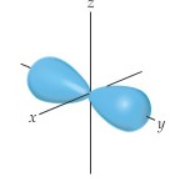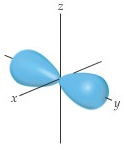# Problem: The contour representation of one of the orbitals for the n = 3 shell of a hydrogen atom is shown in the figure below.What is the quantum number ell for this orbital?

🤓 Based on our data, we think this question is relevant for Professor Meier's class at UNG.

###### FREE Expert Solution

We are asked to identify the quantum number for this orbitalRecall that the quantum numbers that define an electron are:

• Principal Quantum Number (n): deals with the size and energy of the atomic orbital. The possible values for n are 1 to ∞.

• Angular Momentum Quantum Number (l): deals with the shape of the atomic orbital. The possible values for l are 0 to n – 1.

• Magnetic Quantum Number (ml): deals with the orientation of the atomic orbital in 3D space. The possible values for ml are the range of –l to +l.

• Spin Quantum Number (ms): deals with the spin of the electron. The possible values for ms are either –1/2 (spin down) or +1/2 (spin up).###### Problem Details

The contour representation of one of the orbitals for the n = 3 shell of a hydrogen atom is shown in the figure below.What is the quantum number for this orbital?